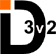com.beaglebuddy.id3.pojo.v23

Class Level

• java.lang.Object
• com.beaglebuddy.id3.pojo.v23.Level

• ```public class Level
extends java.lang.Object```A Level is a point on an EQ curve. It contains the following fields.

Level Fields
FieldDescription
1. `direction`whether the point on the EQ curve is above or below the midpoint.
2. frequency the frequency (in hz) at which the point on EQ curve is located.
3. adjustment the amount above/below the midpoint where the point lies on the EQ curve.

• Nested Class Summary

Nested Classes
Modifier and Type Class and Description
`static class ` `Level.Direction`
indicates whether an equalization level is above or below the midline.
• Constructor Summary

Constructors
Constructor and Description
`Level()`
The default constructor is called when creating a flat level.
```Level(Level.Direction direction, int frequency, int adjustment)```
constructor.
• Method Summary

Methods
Modifier and Type Method and Description
`int` `getAdjustment()`
get the amount of adjustment for the equalization level.
`Level.Direction` `getDirection()`
get the direction of the equalization level, ie whether it is above or below the midline.
`short` `getFrequency()`
get the frequency (in hz) at which the equalization level occurs.
`void` `setAdjustment(int adjustment)`
set the amount of adjustment for the equalization level.
`void` `setDirection(Level.Direction direction)`
sets the direction of the equalization level, ie whether it is above or below the midline.
`void` `setFrequency(int frequency)`
set the frequency (in hz) at which the equalization level occurs.
`java.lang.String` `toString()`
get a string representation of an equalization level.
• Methods inherited from class java.lang.Object

`clone, equals, finalize, getClass, hashCode, notify, notifyAll, wait, wait, wait`
• Constructor Detail

• Level

`public Level()`
The default constructor is called when creating a flat level. The default values used are:
• INCREMENT
• 0 hz
• amount of adjustment set to 1
• Level

```public Level(Level.Direction direction,
int frequency,
constructor.
Parameters:
`direction` - increment or decrement, ie, above or below the midline.
`frequency` - frequency (in hz)
`adjustment` - adjustment from 0.
• Method Detail

• setDirection

`public void setDirection(Level.Direction direction)`
sets the direction of the equalization level, ie whether it is above or below the midline.
Parameters:
`direction` - the direction of the equalization level.
`getDirection()`
• getFrequency

`public short getFrequency()`
get the frequency (in hz) at which the equalization level occurs.
Returns:
the frequency (in hz) at which the equalization level occurs.
• setFrequency

`public void setFrequency(int frequency)`
set the frequency (in hz) at which the equalization level occurs.
Parameters:
`frequency` - the frequency (in hz) at which the equalization level occurs.
Throws:
`java.lang.IllegalArgumentException` - if the specified frequency is less than 0 hz or greater than or equal to 32768 hz.

`public int getAdjustment()`
get the amount of adjustment for the equalization level.
Returns:
the amount of adjustment for the equalization level.

`public void setAdjustment(int adjustment)`
set the amount of adjustment for the equalization level.
Parameters:
`adjustment` - the amount of adjustment for the equalization level.
Throws:
`java.lang.IllegalArgumentException` - if the adjustment less than or equal to 0.
• toString

`public java.lang.String toString()`
get a string representation of an equalization level.
Overrides:
`toString` in class `java.lang.Object`
Returns:
a string representation of an equalization level.